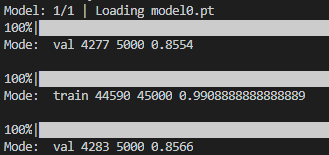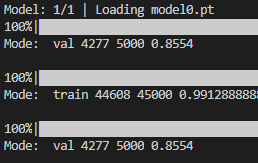# Strange behaviour in PyTorch

I have a classifier, and I want to compute outputs from the model in the training and validation modes. I am also checking their respective accuracies. However, I found one interestingly strange behaviour.

I have already pre-trained the model (ResNet-18) on Cifar-10 and saved the best model on the validation set with accuracy 85.54%. When I run `get_outputs` function on the same data, I get same accuracy in the val set. However, if I will change the order of mode, s.t. instead of `for mode in ['val', 'train']` I will write `for mode in ['train', 'val']`, val accuracy will be 85.66%. I do not understand why this happens.

``````@torch.no_grad()
batches = {'train': [], 'val': []}
correct = {'train': 0, 'val': 0}
total = {'train': 0, 'val':0}
for params in model.parameters():

for mode in [ 'val', 'train']:
if mode == 'train':
model.train()
else:
model.eval()
for _, (inputs, targets) in enumerate(tqdm(loader_dict[mode])):
inputs, targets = inputs.to(device), targets.to(device)
outputs = model(inputs)
batches[mode].append(outputs.detach().cpu())
_, predicted = outputs.max(1)
predicted = predicted.cpu()
total[mode] += targets.size(0)
correct[mode] += predicted.eq(targets.cpu()).sum().item()
return correct, total
``````

The way I create a `loader_dict`:

``````	train_size = int(0.9 * len(dataset))
val_size = len(dataset) - train_size
train_data, val_data = random_split(dataset, [train_size, val_size], generator = torch.Generator().manual_seed(42))

num_workers = 8, pin_memory = True, generator = torch.Generator().manual_seed(42))
num_workers = 8, pin_memory = True, generator = torch.Generator().manual_seed(42) )

``````

In the picture above you can see that if mode `'val'` is before `'train'`, then the number of correct images is 4277. However, when the mode `'val'` is after `'train'`, then the number of correct images is 4283. As for the training set, they are same.

@ptrblck could you look at this?

I printed the squared output of the val set in different orders.

When `'val'` follows `'train'` the squared output is 4932.34765625. Otherwise it is 4933.31591796875.

Swapping the training and validation loop might change the order or calls into the pseudorandom number generator and might thus yield a different final accuracy.
You could rerun both approaches with different seeds and check this behavior.

1 Like

I am seeding everything as follows:

``````	torch.manual_seed(42)
torch.cuda.manual_seed(42)
torch.cuda.manual_seed_all(42)
``````

Furthermore, if I will put it like this `for mode in ['val', 'val']`. The answer will be 85.54% each time

However, setting it like this: `for mode in ['val', 'train', 'val']` will yield different results, 85.54% and 85.66% respectively.

Choosing different seeds doesn’t change the behavior.

I think I found the mistake.

When I use `for mode in ['val', 'train', 'val']` accuracies are different during validation sets, 85.54% and 85.66%. However, If I will remove `model.train()`, everything works fine. Thus, I think that `model.train()` affects the network. I checked the value of parameters, and they are identical. I think that somehow dropout layer is affected after we do `model.train()`

In training mode dropout layers will be activated and batchnorm layers will normalize the input batch using the batch statistics and will also update their internal running stats.
I’m not sure where you’ve called `.train()` and `.eval()`, but these operations should be called in the corresponding training and evaluation loops, respectively.

1 Like

In the first post I showed how I apply `model.eval()` and `model.train()`. Below what happens when I loop the mode over `['val', 'train', 'val']`:When I remove `mode.train()` and there will be only `model.eval()`. The result is following:The rightmost values are accuracies.

Interestingly when I put it like this:

``````for mode in ['val', 'train', 'val', 'train', 'val']:
``````

It returns 85.54%, 85.66% and 85.44%. I am not sure what is happening. But anyway, thanksCould you look at it? @albanD @smth @tom

Thanks @ptrblck and @tom! I just understood it